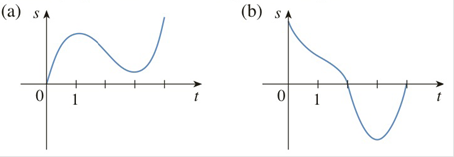Chapter 2.7, Problem 6E

Chapter
Section
Textbook Problem

# Graphs of the position functions of two particles are shown, where t is measured in seconds. When is each particle speeding up? When is it slowing down? Explain.To determine

Part (a)

To explain: When is each particle speeding up and speeding down

Explanation

Velocity is rate of change of position with respect to time that is velocity is the derivative of position of the particle

From the above graph velocity is positive on the interval (0, 1) and (3, 4), since the slope is positive.And velocity is negative on the interval (1, 3) Since the slope is negative

Now, Acceleration is rate of change of velocity with time that is acceleration is derivative of velocity.Acceleration is positive on the interval (2, 4) since the graph is concave up

And negative on the interval (0, 2) since the graph is concave down

To determine

Part (b)

To explain: When is each particle speeding up and speeding down

### Still sussing out bartleby?

Check out a sample textbook solution.

See a sample solution

#### The Solution to Your Study Problems

Bartleby provides explanations to thousands of textbook problems written by our experts, many with advanced degrees!

Get Started

#### 16x4y84

Applied Calculus for the Managerial, Life, and Social Sciences: A Brief Approach

#### Multiply: 3709731

Elementary Technical Mathematics

#### (y)2 + 6xy 2y + 7x = 0 is a differential equation of order ______. a) 1 b) 2 c) 3 d) 4

Study Guide for Stewart's Single Variable Calculus: Early Transcendentals, 8th